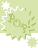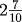Pop-ups: Choose the correct answer from a list of choices.# Express the decimal as a fraction

A decimal is another expression of a fraction. 4.25 is 4 and 25 hundredths. or(of course reduce). 2.7 is 2 and 7 tenths or. The decimal part represents the fraction. What is the digits place of the last decimal? Tenths, express the decimal value over 10, Thousandths? Express the decimal value over 100.

Express as an improper fraction! An unreduced answer is a wrong answer!This activity was created by a Quia Web subscriber. Learn more about QuiaCreate your own activities